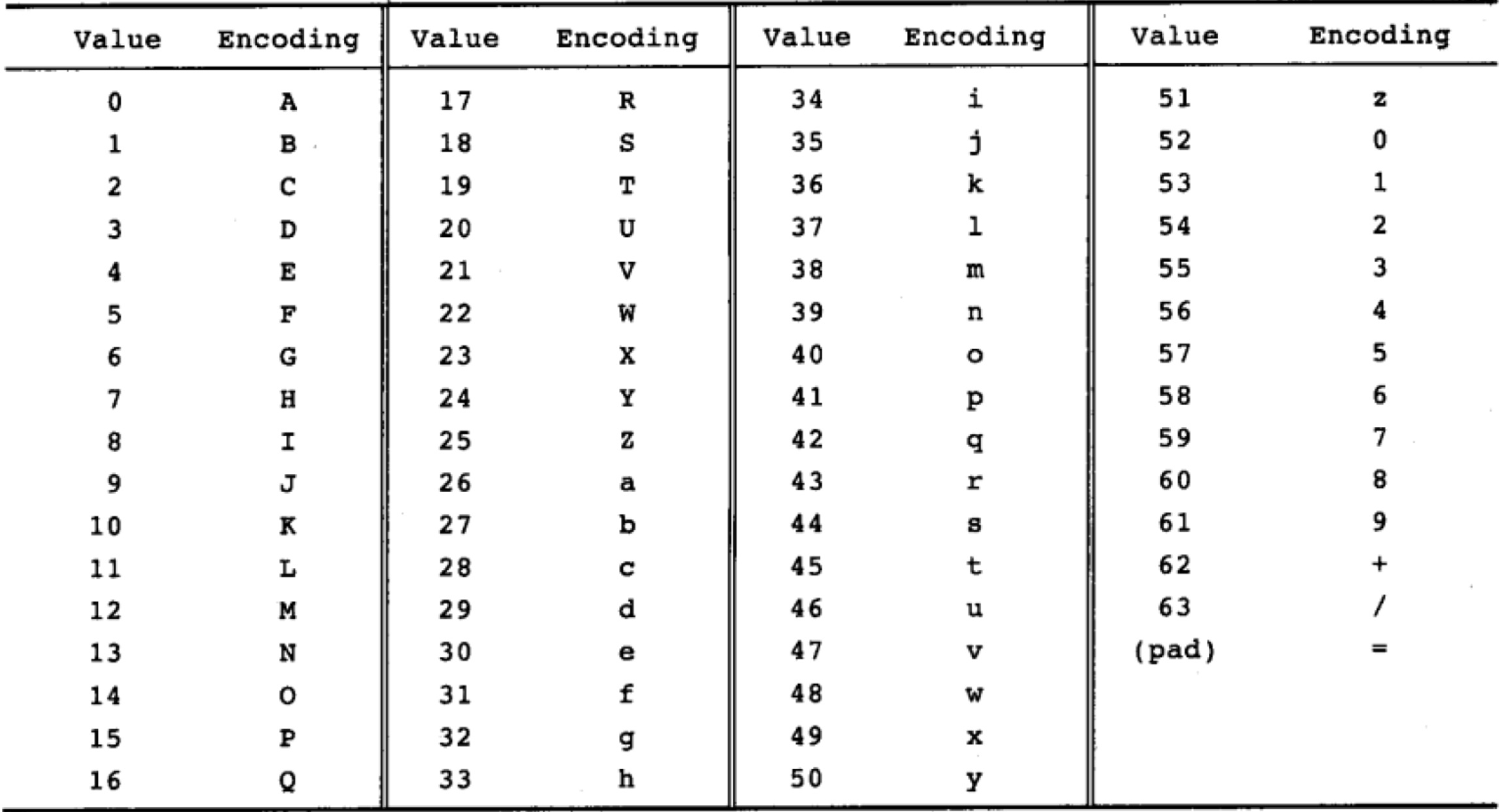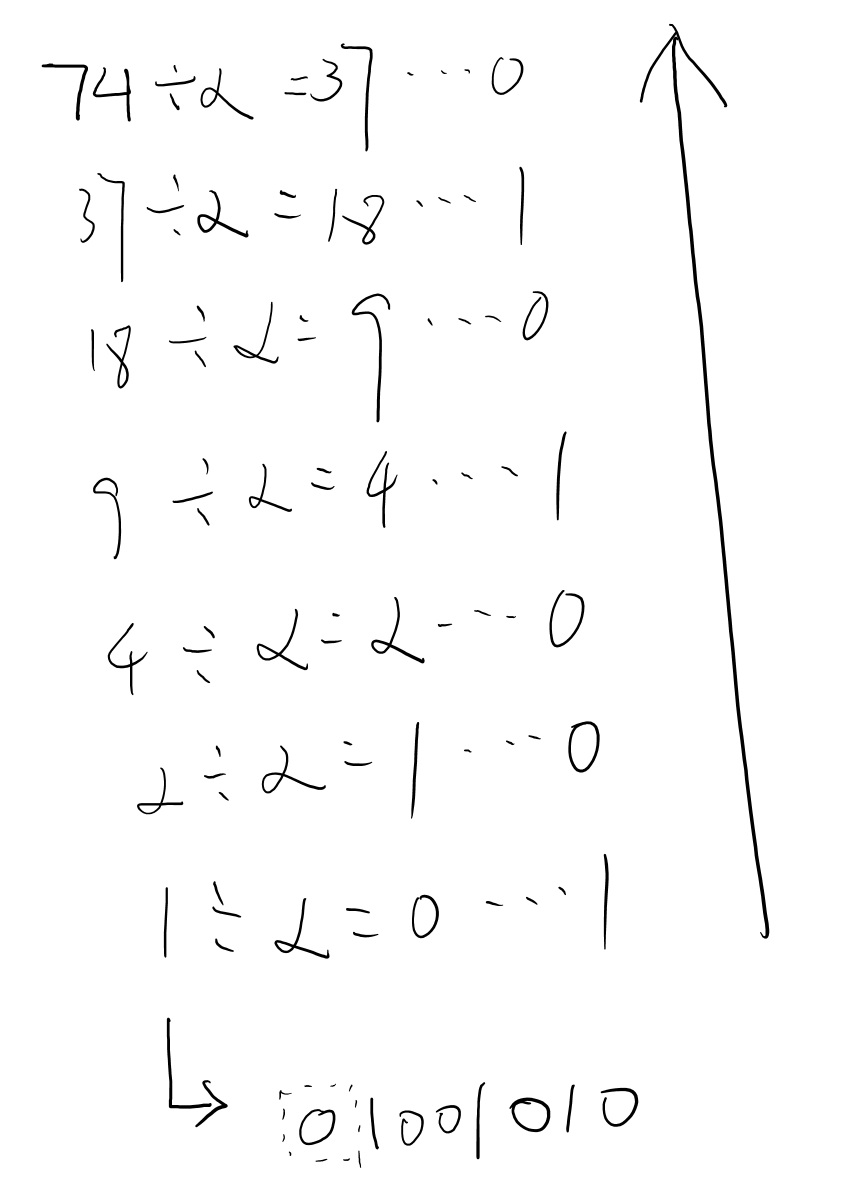# The Base64 algorithm in action

First we have a base64 mapping table, like below:This is used to get the character for the base64 encrytion.

Now, we try to manually calculate the `java`.

### Get UTF-8 ASCII code

``````package encryption;

import java.io.UnsupportedEncodingException;

/**
* encryption.Base64Test
* <p>
* Author: ChrisYe
* Date: 10/8/2017
*/

public class Base64Test {

public static void main(String... arg) throws UnsupportedEncodingException {
String testVal = "Java";

StringBuilder sb = new StringBuilder();
for(byte b : testVal.getBytes()){
sb.append(Integer.toString(b)).append(" ");
}
System.out.println(sb.toString());
}

}
``````

So we get the code below:

``````74 97 118 97
``````

### Then we turn the Decimal System to Binary systemCalculate step by step, then we get:

``````01001010 01100001 01110110 01100001
``````

### Turn the Binary system to Base64 codePS:

1. 6 bit for one base64 code
2. Must end with 24 bit
3. Less then 6 bit, low fill with 0
4. Less then 24, low fill with =

So the Final base64 encrption string is: `SmF2YQ==`